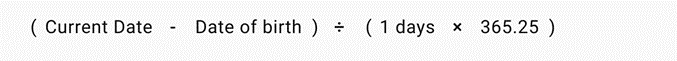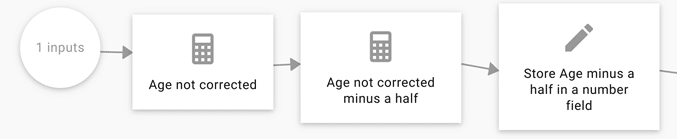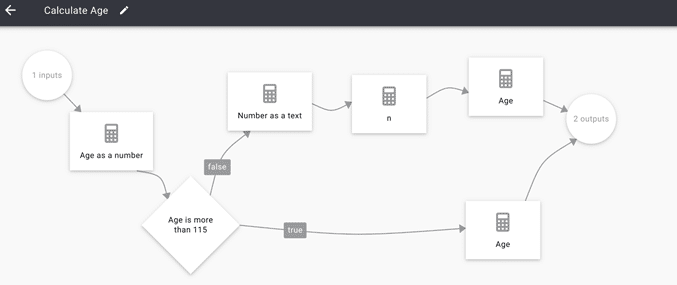# How to calculate age

The formula to calculate age is: (Current date – Date of birth) / 1 year.Triggre does not know ‘year’, so you have to use 365.25 * 1 day. This takes account of leap years.The result is a number with six decimals. You must reduce that to the integer value: 46.653876 must become 46.

There are two ways to do this:

1. Store the outcome in a property of the type Number with 0 decimals.
Do this if you want to use Age in calculations or overviews.
2. Convert the outcome to a text.
Do this if you don’t want to store Age for later use, but want to use it in an email or on a page in a User flow.

## Store the age in a data item as a number

Triggre will round the outcome up or down, depending on the value.  But, when calculating age, you always want to round down. For example, 46.7 should become 46, not 47.

Rounding down a number
To make Triggre round numbers down, you subtract 0.5 from the value before you store it.

Explanation
Let’s use the age of 46 as an example. The smallest possible result of the function is 46.000000, the largest is 46.999999.

When you subtract 0.5, they will become 45.500000 and 46.499999.

When you store these values or any value in between, Triggre will round them to 46.

Tip: Be aware that when you store the age in a data item, you periodically have to check whether the age has changed.## Convert the outcome to a text

1. Convert the number to a text:
(combine with an empty Specific value.)2. Remove the decimal point and everything to the right of it.
This is explained in the article Conversions between numbers, texts and dates.
3. Include the age in the output.

## Flow parts

Included is a decision to validate the date of birth. If it’s empty or > 115 years ago, the output will be a message suggesting a better input.